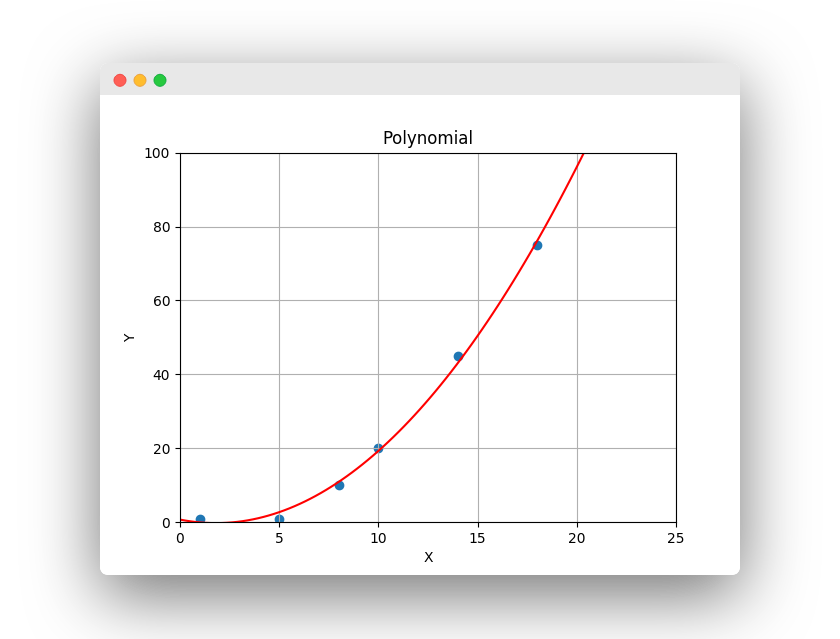Polynomial regression can be very useful. There isn’t always a linear relationship between X and Y. Sometime the relation is exponential or Nth order.

Related course: Python Machine Learning Course

## Regression

### Polynomial regression

You can plot a polynomial relationship between X and Y. If there isn’t a linear relationship, you may need a polynomial. Unlike a linear relationship, a polynomial can fit the data better.You create this polynomial line with just one line of code.

That would train the algorithm and use a 2nd degree polynomial.
After training, you can predict a value by calling polyfit, with a new example. It will then output a continous value.

### Example

The example below plots a polynomial line on top of the collected data. It trains the algorithm, then it makes a prediction of a continous value.

### Overfitting and underfitting

It’s important to not overfit or underfit, you want to capture the relationship but not follow the points exactly. A linear relationship would underfit, overfitting would be picking the degree so high that it fits the points. Instead, you want to capture the relationship.

If you are new to Machine Learning, then I highly recommend this book.